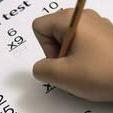3 math skills every student should have
FRIDAY, APRIL 10, 2015 10:20 AMThe Common Core State Standards are shifting the way math is taught by emphasizing fluent knowledge and using problems with more complexity. In this way, the Standards are aiming for math education that's less about memorizing equations and more about using independent reasoning to solve complex issues. In fact, the Common Core defines mathematical standards that students at every grade level should be able to use to solve problems by themselves. Here are three of those standards that can help students build fluency and mathematical independence:

1. Reasoning
The Standards state that students should be able to use both quantitative and abstract reasoning skills in order to understand how to solve a problem or which computations should be used. One way a State Standards test might encourage students to reason is by giving them a problem without providing the equation they need to solve it. In past tests, students may have seen a question like this: "Find the length of one side of this triangle using the Pythagorean theorem, where a²+b²=c²." In that case, students would simply have to plug the numbers given to them into the equation and solve for the final variable. The Common Core, however, might ask the same question without providing the formula. At that time, students would have to first reason the best way to solve the problem, and then use that reasoning to come up with a final answer.

2. Perseverance
Another key skill of practicing mathematics involves using perseverance in solving complex problems. In other words, students might be asked to solve a difficult or multi-faceted problem that they may not comprehend immediately or that might require multiple computations. Students should know how to use the knowledge they have to break a problem down into more manageable and understandable steps, and solve it accordingly. This also requires a fluent understanding of mathematical concepts as well as the ability to independently reason.

3. Modeling
When it comes to teaching math, one of the best ways instructors can keep students engaged and excited is by connecting mathematics and statistics to real-world problems. The Common Core calls this practice "modeling," and expects students to be able to solve real?-world problems mathematically as they advance through middle and high school. For instance, they may be asked to use geometric and algebraic standards to figure out how much fertilizer is necessary to prep the soil of a farmer's field.RELATED ARTICLES All About TerraNova 3 Testing Teaching gifted kids in normal classes Helping your kids continue learning this summer More..
 NEWS CATEGORIES NEWS ARCHIVE